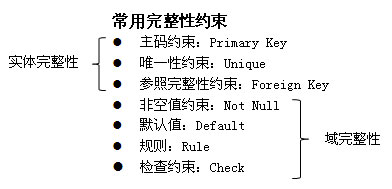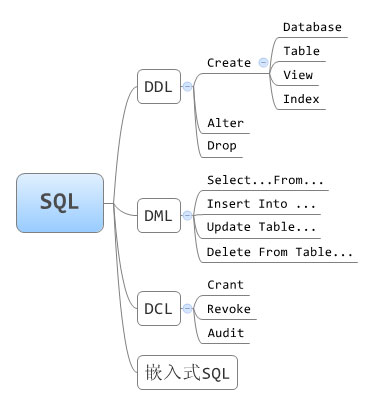# SQL Server中常用的SQL语句

### 1、概述

#### 数据完整性

• 实体完整性：主属性不能为空值，例如选课表中学号和课程号不能为空
• 参照完整性：表中的外键取值为空或参照表中的主键
• 用户定义完整性：取值范围或非空限制，例如：性别（男女），年龄（0-130）#### 表连接

• 自然连接：与等值连接（a.id=b.id）相比，连接后的表只有一列id，而不是两列（a.id和b.id）。
• 半连接：与等值连接（a.id=b.id）相比，连接后的表只有A表的列，被B表“多次匹配”列会显示为一行。
• 左外连接：left join
• 右外连接：right join
• 全外连接：full join
• 全内连接：inner join

#### SQL语言的构成

• DDL语言：数据定义，定义基本表、视图、索引；
• DML语言：数据操纵，查询、增加、修改、删除
• DCL语言：权限### 2、查询概述

```select…            ⑤    投影
from…              ①    table→内存
where…             ②    选取元组
group…             ③    分组
having…            ④    选择分组
[{union|…}         ⑥    查徇结果的集      合运算
select…  ]         ①～⑤
order by…          ⑦    排序输出
```

### 3、单表查询

group by 只有出现在group by子句中的属性，才可出现在select子句中。

having 与 where的区别

• where 决定哪些元组被选择参加运算，作用于关系中的元组
• having 决定哪些分组符合要求，作用于分组

### 4、连接查询

```[表名1.] 列名1 比较运算符  [表名2.]列名2
```

```[表名1.]列名1  between [表名2.]列名2 and [表名2.]列名3
```

#### 4.1 单表连接（自连接）

```select FIRST.sno
from SC as FIRST, SC as SECOND
where FIRST.Sno=SECOND.Sno and FIRST.cno='s2' and SECOND.cno='4'
```

#### 4.3 嵌套查询

• 在select … from … where语句结构的where子句中可嵌入一个select语句块
• 其上层查询称为外层查询或父查询，其下层查询称为内层查询或子查询
• SQL语言允许使用多重嵌套查询
• 在子查询中不允许使用order by子句
• 嵌套查询的实现一般是从里到外，即先进行子查询，再把其结果用于父查询作为条件
##### 4.3.1 返回单个值的子查询

```方法一：
select Sname, Sage
from student
where Sdept = (select sdept from student where  Sname = "刘力");

select FIRST.Sname, FIRST.Sage
from Student FIRST, Student SECOND
where FIRST.Sdept = SECOND.Sdept AND SECOND.Sname = "刘力";
```
##### 4.3.2 返回一组值的子查询

```方法一：
select * from
student
where sno IN (select sno from SC where Cno="C6" AND Grade>90);

select student.*
from student，SC
where Student.Sno=SC.Sno AND Cno="C6" AND Grade>90;
```

```方法一：
select *
from student
where
sdept!="CS" AND
sage < ANY (select Sage from Student where Sdept="CS");

select  *
from Student
where
Sdept!=’CS’ AND
Sage < (select MAX(Sage) from Student where Sdept="CS");
```
##### 4.3.3 多重子查询

```表结构：
Teacher(tno, tname, salary, dno)
Department(dno, dname)

select Tname，Salary
from Teacher
where
Dno = "D01" AND
salary IN(
select salary from  teacher
where Dno =（select DNO from department where  Dname="国贸")
);
```

```select *
from teacher
where
Salary >= (select MIN(Salary) from teacher where Tname IN ("张三", "里司")) AND
Salary <= (select MAX(Salary) from teacher where Tname IN ("张三", "里司");
```
##### 4.3.4 在from语句中使用子查询，对查询结果定义表名及列名

```select Sno, avg_G
from (select Sno, avg(Grade) from SC group by Sno) AS RA(Sno, avg_G)
where avg_G > 80;
```

AS RA(Sno, avg_G)，为查询作为定义表名（RA）和列名（Sno, avg_G）

### 5、带有exists的相关子查询

• 不相关子查询：子查询的查询条件不依赖于父查询的称为不相关子查询。
• 相关子查询：子查询的查询条件依赖于外层父查询的某个属性值的称为相关子查询，带exists 的子查询就是相关子查询
• exists表示存在量词，带有exists的子查询不返回任何记录的数据，只返回逻辑值“True” 或“False”

```不相关子查询：
select Sname from student where sno IN ( select sno from SC where Cno = "C1" );

select Sname from student
where exists (select * from SC where student.sno=SC.sno AND Cno = "C1" );
```

• 不关心子查询的具体内容，因此用 select *
• exists + 子查询用来判断该子查询是否返回元组
• 当子查询的结果集非空时，exists 为“True”；当子查询的结果集为空时，exists为“False”。
• not exists ：若子查询结果为空，返回“TRUE”值，否则返回“FALSE”

```select Sname
from student
where not exists (
select *
from Course
where not exists (
select * from SC where
student.sno=SC.sno AND Course.Cno=SC.Cno
)
);
```

```select Sname
from student
where not exists(
select *
from SC SCX
where
SCX.sno="s1" AND
not exists (
select *
from SC SCY
where student.sno=SCY.sno AND SCX.Cno=SCY.Cno
)
);
```

### 6、SQL的集合操作

• 属性个数必须一致、对应的类型必须一致
• 属性名可以不一致， 最终结果集采用第一个结果的属性名
• 缺省为自动去除重复元组，除非显式说明ALL
• order by放在整个语句的最后

#### 6.1 “并”操作，例：查询计算机系的学生或者年龄不大于19岁的学生，并按年龄倒排序。

```select * from student where Sdept="CS"
UNION
select * from student where AGE <= 19
order by AGE desc
```

#### 6.2 “交”操作，例：查询计算机系的学生并且年龄不大于19岁的学生，并按年龄倒排序。

```(select * from student where Sdept = "CS")
INTERSECT
(select * from student where AGE <= 19)
order by AGE desc
```

#### 6.3 “差”操作，例：查询选修课程1但没有选修课程2的学生。

```select Sname, Sdept
from student
where sno IN (
(select sno from SC where Cno="1")
EXCEPT
(select sno from SC where Cno="2")
)
```

### 7、插入操作

```格式：insert  into  表名[(列名1,…)]  values  (列值1,…)
```

#### 插入一已知元组的全部列值

```insert into student values("2003001", "陈冬", 18, "男", "电商", "管理学院", "徐州");
```

#### 插入一已知元组的部分列值

```insert into SC(Sno，Cno) values ("2003001", "C003");
```

#### 插入子查询的结果

##### 例：设关系S_G(Sno,avg_G),把平均成绩大于80的男生的学号及平均成绩存入S_G中
```insert into S_G(sno，avg_G) (
from SC
where Sno IN (select Sno from Student where SEX="男")
group  by  Sno
);
```

### 8、删除操作

```格式：
delete from 表名 [where 条件];
```
• 只能对整个元组操作，不能只删除某些属性上的值
• 只能对一个关系（表）起作用，若要从多个关系（表）中删除元组，则必须对每个关系分别执行删除命令

### 9、修改操作

update语句一次只能操作一个表。

```格式1：
update 表名 [别名]
set 列名 = 表达式, ...
[where 条件];

update 表名 [别名]
set (列名, ...) = (子查询)
[where 条件];
```

#### 例：工种为SALESMEN的职工的工资改为工种平均工资的110%

```update EMPLOYEE
set Salary = (select 1.1 * avg(Salary) from EMPLOYEE where JOB="SALESMEN")
where JOB="SALESMEN";
```

#### 例：将所有学生的年龄增加1岁

```update student set Sage=Sage+1;
```

### 10、数据定义

#### 创建课程表

```create table SC (
sno CHAR(6) not null,
Cno CHAR(6) not null,
)
primary key (sno,Cno)
foreign key (sno) references student(sno)
foreign key (Cno) references Course(Cno)
check (Grade between 0 AND 100);
```

#### 常用的索引:唯一索引和聚簇索引

##### 唯一索引
• 对于已含重复值的属性列不能建UNIQUE索引
• 对某个列建立UNIQUE索引后，插入新记录时DBMS会自动检查新记录在该列上是否取了重复值。这相当于增加了一个UNIQUE约束
```create UNIQUE INDEX  Stusno ON Student(Sno ASC);
```
##### 聚簇索引

```create CLUSTER INDEX Stusname ON Student(Sname);
```

• 在一个基本表上最多只能建立一个聚簇索引
• 聚簇索引的用途：对于某些类型的查询，可以提高查询效率
• 聚簇索引的适用范围：很少对基表进行增删操作；很少对其中的变长列进行修改操作
##### 删除索引

```DROP INDEX [表名.]<索引名>;
```

```DROP INDEX Student.Stusname;
```

### 11、视图

```create view ec_student
as
select sno, sname, age from student where dept="ec"
```

```DROP VIEW <视图名>
```

• 暂无评论